Algebra

Equations

Inequalities

Graphs

Numbers

Calculus

Matrices

Tutorials

# Multiplication of Polynomials

Multiplication of Polynomials

Deﬁnition and Notation

The product of two natural numbers, 3and 4, is deﬁned by

3 x 4 = 4+4+4 three terms of 4
Similarly   5 a = 5·a = a+a+a+a+a ﬁve terms of a
4 ab = ab+ab+ab+ab four terms of ab
ab=a x b = b+b +....+ b a terms of b

The following are some of the laws from multiplication of real numbers

1. The commutative law of multiplication: ab = ba

2. The associative law of multiplication: a (bc) = (ab) c

3. The distributive law of multiplication: a (b+c) = (b+c) a

=ab+ac

4. Multiplication of signed numbers:

(+ a) (+ b) = + ab; (+ a) ( b) = ab

( a) (+ b) = ab; ( a) ( b) = + ab

When we have 2·2·2·2. that is, four factors of 2, the notation 24 is used, which reads. “two to the power four," or “two to the fourth power."

Similarly, a·a·a·a·a = a5 means ﬁve factors of a. The a is called the base, and the 5 is called the exponent. When there is no exponent, as in x, we always mean x to the power 1.

DEFINITION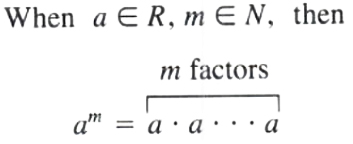Note the difference between

( 24) = ( 2) ( 2) ( 2) ( 2) = +16

and 24 = (24) = -(2·2·2·2) = 16

Note also 2 a3 = 2 (a·a·a)

While (2 a)3 = (2 a) (2 a) (2 a)

=(2·2·2) (a·a·a)

=23 a3 = 8 a3

Remark a , a2 , a3,.... are not like terms.

EXAMPLES 1. 7 a·a·a·a = 7 a4

2. ( 3) ( 3) ( 3) ( 3) = ( 3)4

3. (x-1)3 = (x-1) (x-1) (x-1)

4. 22·33 = (2·2)·(3·3·3) = 4·27 = 108

5. 22+23 = 2·2+2·2·2 = 4+8 = 12

6. 23-2 = 2·2·2-2=8-2 = 6

Multiplication of Monomials

We will discuss the multiplication of monomials, then the multiplication of a monomial and a polynomial, and ﬁnally the multiplication of two polynomials.

From the deﬁnition of exponents we have

a3·a5 = (a·a·a) (a·a·a·a·a)

=a·a·a·a·a·a·a·a

=a8

=a3+5

THEOREM 1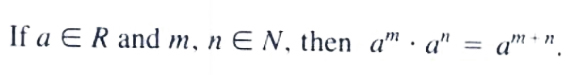ProofEXAMPLES 1. 23·25 = 23+5 = 28

2. a2·a4 = a2+4 = a6

3. 24·23 = 24+3 = 27

4. 3 x3·x2 = 3 x3+2 = 3 x5

5. x5·x = x5+1 = x6

6. (a+1)2·(a+1)3 = (a+1)2+3 = (a+1)5

Let's see how our Polynomial solver simplifies this and similar problems. Click on "Solve Similar" button to see more examples.

Remark 23·27 = 23+7 = 210 , and not 410

Remark 24·35 = 24·35 , to find the product, multiply 24 =16 by 35 =243 ; that is 24·35 = (16) (243) = 3888

Since the commutative and associative laws for multiplication hold for numbers, speciﬁc or general. we have

EXAMPLES 1. (2 ab2) (3 a4 bc2)= (2·3) (a1·a4) (b2·b1) (c2)

= 6 a5 b3 c2

2. ( 3 b2 c3) (8 ab3 c) = ( 3·8) (b2·b3) (c3·c) (a)

= 24 b5 c4 a

3. ( 32 xy2) ( 5 x2 y3) = ( 9 xy2) ( 5 x2 y3)

=( 9) ( 5) (x·x2) (y2·y3)=45 x3 y5

Let's see how our Polynomial solver simplifies this and similar problems step-by-step. Click on "Solve Similar" button to see more examples.

From the deﬁnition of exponents we have

(a2)3 = (a2) (a2) (a2)

=(a2·a2) (a2)

=a2+2·a2

=a2+2+2

=a3·2 = a2·3

=a6

THEOREM 2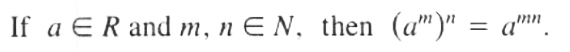Proof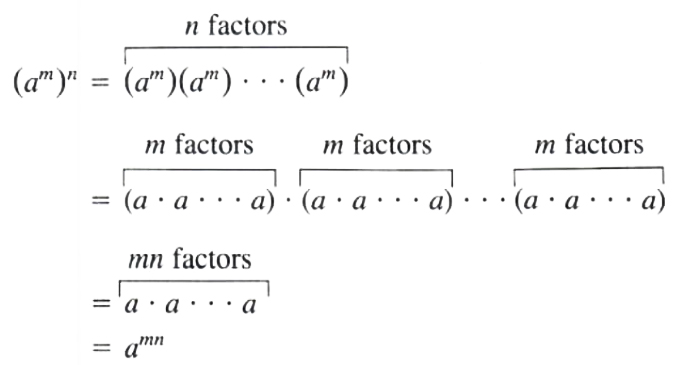EXAMPLES 1. (32)4 = 32·4 = 38

2. (a3)5 = a3·5 = a15

3. ( 32)3 = 32·3 = 36

4. ( a3)2 = a3·2 = a6

Note 23·24 = 23+4=27, While (23)4 = 23·4=212

From the deﬁnition of exponents we have

64 = (2·3)4 = (2·3) (2·3) (2·3) (2·3)

= (2·2·2·2) (3·3·3·3)

=24·34

THEOREM 3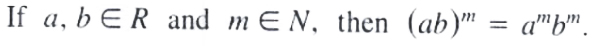Proof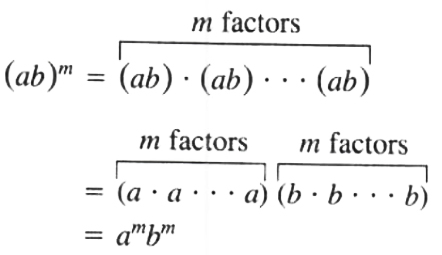Note a and b are factors. If a=3, b=x, and m=5,(3 x)5=35 x5

Do not forget to raise the number 3 to the power 5

Applying Theorem 3 repeatedly, we obtain

(abcd)m=[(ab) (cd)]m

=(ab)m (cd)m

=ambmcmdm

Remark 212 = (3·7)2 = 32·72 = 9 x49 = 441

Remark The quantity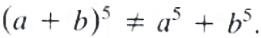(5+3)2 = (8)2 = 64, but 52+32 = 25+9 = 34

If we consider (a+b) as one quantity, then

(a+b)5 = (a+b) (a+b) (a+b) (a+b) (a+b)

The method of calculating the product will be explained later

COROLLARY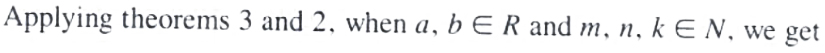(am) (bn)k = [(am) (bn)]k

=(am)k (bn)k

=amkbnk

EXAMPLE Perform the following multiplication: (2 x2 yz3)( 4 x3 y2)

Solution (2 x2 yz3)( 4 x3 y2) = (2) ( 4) (x2·x3) (y·y2) (z3)

= 8 x5 y3 z3

Let's see how our Polynomial solver simplifies this and similar problems, showing explanations for each step. Click on "Solve Similar" button to see more solved examples.

EXAMPLE Perform the following multiplication: (5 a2 b)3

Solution (5 a2 b)3 = (5)3 (a2)3 (b)3 = 53 a6 b3 = 125 a6 b3

EXAMPLE Perform the following multiplication: 22 a3 (ab3)2

Solution 22 a3 (ab3)2 = 4 a3 (a2 b6) = 4 (a3·a2) (b6) = 4 a5 b6

EXAMPLES Perform the following multiplication: (3 x2 y)2 (2 xy3)3

Solution (3 x2 y)2 (2 xy3)3 = (32 x4 y2) (23 x3 y9) = (32·23) (x4·x3) (y2·y9)

=(9·8) x7 y11 = 72 x7 y11

Remark Perform the outside exponents first

EXAMPLE Perform the following multiplication:

( 2 ab2)2 ( 3 a2 b)3 ( bc2)4

Solution ( 2 ab2)2 ( 3 a2 b)3 ( bc2)4 = ( 2)2 a2 b2·( 3)3 a6 b3·( 1)4 b4 c8

=( 2)2 ( 3)3 ( 1)4 (a2·a6) (b4·b3·b4) (c8)

=(4) ( 27) (+ 1) a8 b11 c8

= 108 a8 b11 c8

EXAMPLE Perform the indicated operations and simplify:

(2 ab)4 ( a3 b)2-( 3 a2)3 (a2 b3)2

Solution (2 ab)4 ( a3 b)2-( 3 a2)3 (a2 b3)2 = (16 a4 b4) (a6 b2)-( 27 a6) (a4 b6)

=16 a10 b6+27 a10 b6

=43 a10 b6

Let's see how our Polynomial calculator explains all simplification steps for this and similar problems. Click on "Solve Similar" button to see more examples.

Note: To evaluate expressions involving exponents. ﬁrst replace each letter by its indicated speciﬁc value. Use grouping symbols where necessary so as not to confuse operation signs with number signs.

Evaluate a2 b3, give that a = 3 and b = 2

Solution a2 b3 = ( 3)2 (2)3 = (9) (8) = 72

EXAMPLE Evaluate the expression b2-a2 (c3-b3), give that a = 2 , b = 3, and c = 1

Solution b2-a2 (c3-b3) = (3)2-( 2)2 [(( 1)3-(3)3)]

=9-(+ 4) [( 1)-(27)]

=9-4 ( 1-27)

=9-4 ( 28)

=9+112 = 121

Multiplication of a Polynomial by a Monomial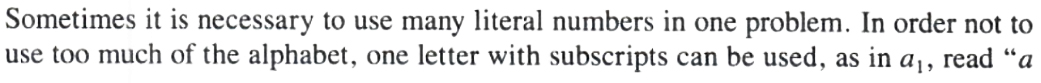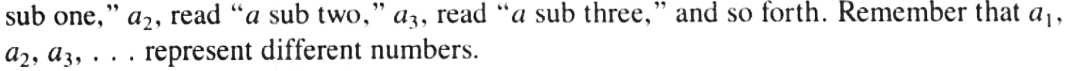The extended distributive law of multiplication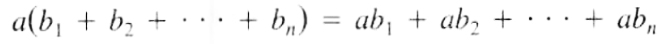is used to multiply a monomial by a polynomial

EXAMPLE Multiply 3 x2+x-2 by x

Solution x (3 x2+x-2) = x (3 x2)+x (x)+x ( 2)

=3 x2+x2-2 x

EXAMPLE Multiply x2-x+4 by 2 x2

Solution ( 2 x2) (x2-x+4) = ( 2 x2) (x2) + ( 2 x2) ( x) + ( 2 x2) (4)

= 2 x4+2 x3-8 x2

EXAMPLE Multiply a2 b-2 b2 c+5 c2 a by 3 a2 b

Solution 3 a2 b (a2 b-2 b2 c+5 c2 a)

=3 a2 b (a2 b)+3 a2 b ( 2 b2 c)+3 a2 b (5 c2 a)

=3 a4 b2-6 a2 b3 c+15 a3 bc2

EXAMPLE Multiply 3 x-24 - 2 x-16 by 12

Solution 121[3 x-24-2 x-16] = 121[3 x-24] - 121 [2 x-16]

=3 (3 x-2)-2 (2 x-1)

=9 x-6-4 x+2

=5 x-4

Multiplication of Polynomials

Multiplication of two polynomials is the same as multiplication of a monomial and a polynomial where the ﬁrst polynomial is considered as one quantity.

To multiply (x+2) by (x-3), consider (x+2) as one quantity and apply the distributive law: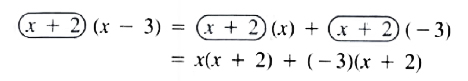Then reapply the distributive law

=x2+2 x-3 x-6

=x2-x-6

Notice that each term of the second polynomial has been multiplied by each term of the ﬁrst polynomial.

The same result can be obtained by arranging the polynomials in two rows and multiplying the upper polynomial by each term of the lower polynomial. Arrange like terms of the product in the same column so that addition is easier.+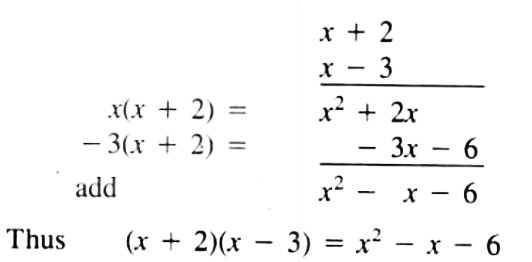EXAMPLE Multiply (3 x-4)2

Solution (3 x-4)2 = (3 x-4) (3 x-4)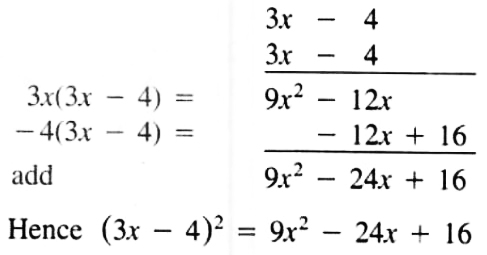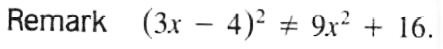Notes 1. (a+b)2 = a2+2 ab+b2

2. (a-b)2 = a2-2 ab+b2

3. (a+b) (a-b) = a2-b2

EXAMPLE Multiply (x2-2 x+1) by (2 x-3)

Solution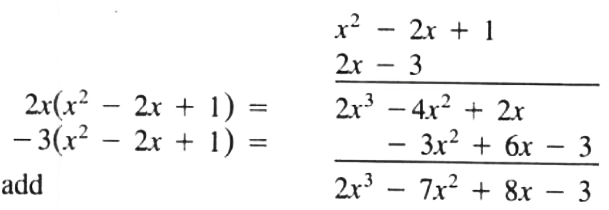Therefore (x2-2 x+1) (2 x-3) = 2 x3-7 x2+8 x-3

EXAMPLE Perform the indicated operations and simplify

(2 x-3) (x+4)-(x+2) (x-6)

Solution (2 x-3) (x+4)-(x+2) (x-6)

=(2 x2-+ 5 x-12)-(x2-4 x-12)

=2 x2+5 x-12-x2+4 x+12

=x2+9 x

Grouping Symbols

Grouping symbols, such as parentheses ( ), braces { }, and brackets [ ], are used to designate, in a simple manner, more than one operation.

When we write the binomial 3 a+5 b as (3 a+5 b), we are considering the sum of 3 a and 5 b as one quantity. The expression a-(b+c) means the sum of b and c is to be subtracted from a.

The statement, three times x minus four times the sum of y and z, can be written in algebraic notation as

3 x-4 (y+z)

Removal of the grouping symbols means performing the operations that these symbols indicate. Remove the symbols one at a time, starting with the innermost, following the proper order of operations to be performed.

EXAMPLE Remove the grouping symbols and combine like terms

2 x-(5 x-2 y)+(x-6 y)

Solution 2 x-(5 x-2 y)+(x-6 y) = 2 x-5 x+2 y+x-6 y

=(2 x-5 x+x)+(2 y-6 y)

= 2 x-4 y

Let's see how our polynomial solver simplifies this and similar problems. Clicking on "Solve Similar" button will show more step-by-step solved examples.

EXAMPLE Remove the grouping symbols and combine like terms

7 a+2 [2 b-3 (3 a-5 b)]

Solution 7 a+2 [2 b-3 (3 a-5 b)] = 7 a+2 [2 b-9 a+15 b]

=7 a+4 b-18 a+30 b

=34 b-11 a

Let's see how our polynomial calculator explains all the steps for this and similar problems. Click on "Solve Similar" button to see more examples solved.

EXAMPLE Remove the grouping symbols and combine like terms

6 a-{2 b+[3-(a+b)+(5 a-2)]}

Solution 6 a-{2 b+[3-(a+b)+(5 a-2)]}

=6 a-{2 b+[3-a-b+5 a-2]}

=6 a-{2 b+3-a-b+5 a-2}

=6 a-2 b-3+a+b-5 a+2

=(6 a+a-5 a)+( 2 b+b)+( 3+2)

=2 a-b-1

Let's see how our polynomial calculator solves this and similar problems. Click on "Solve Similar" button to see more examples.

It is sometimes necessary to group some of the terms of an expression. This can be accomplished by use of a set of parentheses.

When the grouping symbol is preceded by a plus sign. we keep the signs of the terms the same when it is preceded by a minus sign, we use the additive inverses (negatives) of the terms.

EXAMPLE Group the last three terms of the polynomial 3a -  5b + c -  2 with a grouping symbol in two ways, one preceded by a plus sign, the second preceded by a minus sign.

Solution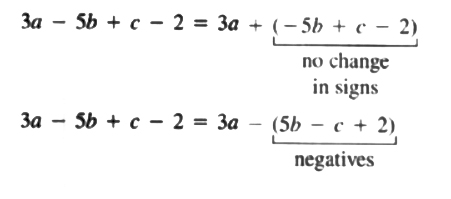← Previous Tutorial Next Tutorial →